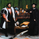# Forecasting - Locally Weighted Regression

1418 просмотров
This is a continuation of the series on forecasting techniques.
Locally weighted linear regression is a non-parametric algorithm, that is, the model does not learn a fixed set of parameters as is done in ordinary linear regression . Rather parameters Θ (theta) are computed individually for each query point x. While computing Θ, a higher “preference” is given to the points in the training set lying in the vicinity of x than the points lying far away from x.
For a detailed discussion see https://www.geeksforgeeks.org/ml-locally...
and for the formula see https://fawda123.github.io/swmp_workshop...

Here you can see a shortcut application of this technique to time series with results unexpectedly favorable for price data labelling.

Good at detecting pullbacks. Can be incorporated into a trading system as a signal generator. Alerting is included.
Скрипт с открытым кодом

In true TradingView spirit, the author of this script has published it open-source, so traders can understand and verify it. Cheers to the author! You may use it for free, but reuse of this code in a publication is governed by House Rules. You can favorite it to use it on a chart.

Хотите использовать этот скрипт на графике?

## Комментарииmercacoin
@mercacoin, see here
scaleMinimax(X, p, min, max) =>
hi = highest(X, p), lo = lowest(X, p)
(max - min) * (X - lo)/(hi - lo) + min

scale(X, p) => scaleMinimax(X, p, lowest(close, p), highest(close, p))
Ответитьgreyghost7
@greyghost7, it uses the current value of the price, not the previous.
ОтветитьUPD: here's the mod that allows to work with securities other than BTC. Replace the lines 19 thru 35 with the following:

locally_weighted(x, p, b, mx) =>
PI = 3.14159265359
l = 0.0
for i=0 to p-1
l := b + b*i + b*2*x + b*sin(2*PI*i) + b*cos(2*PI*i)
scale(linreg(l, p, 0), mx)

//*** Inputs
price = input(close, "Price Data")
p = input(5, "Lookback Window")
span = input(8, "Rising/Falling span", minval=2)
crvs = input(false, "Show curves?")
lbls = input(true, "Show Labels?")
mmx = input(2, "Scaler Lookback", minval=1)

//*** Main
BETA = .5
lwr = locally_weighted(price, p, BETA, mmx)
Ответить
Профиль Настройка профиля Счёт и оплата Ваши друзья Монеты Мои запросы в поддержку Справочный центр Личные сообщения Чат Выйти